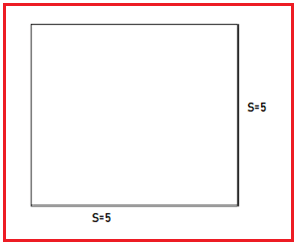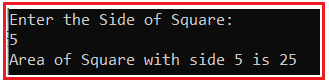# Area of Square in C#

## Area of Square Program in C# with Examples

In this article, I am going to discuss how to calculate the Area of a Square in C# with Examples. Please read our previous article, where we discussed the Area of Rectangle Program in C#. Here, in this article, first, we will discuss what is Square, then we will discuss how to calculate the area of a square and finally, we will see how to implement the area of the square program in C#.

##### What is a Square?

According to Wikipedia, in geometry, a square is a regular quadrilateral, which means that it has four equal sides and four equal angles (90-degree angles, or 100-gradian angles or right angles). It can also be defined as a rectangle in which two adjacent sides have equal lengths. A square is a closed, two-dimensional shape with 4 equal sides and four right angles.

##### How to Calculate the Area of a Square?

Area of square = side * side Or
A=S * S, where, A is the area of the square and S is the side of the square

For example: Let say S i.e. side = 5, then the area of the square is 25 i.e. 5 * 5
Explanation: area of the square is the multiply of side with sideIn this program, we can use the following Datatype

1. int: Integer variables are variables that must take an integer value (0, 1, 2, …). Example: variables int num1 = 5
2. float: Floating-point numbers are used for decimal and exponential values. It is a primitive data type. Example: variables float num1 = 5.5
##### Logic to Calculate the Area of Square in C#

For finding the area of the square we will multiply simply side with itself. The following are the steps that we need to follow to solve this problem.

1. First, we will define side S.
2. Then define the area of square A.
3. Then calculate the area of the square by multiplying side(S) with side(S).
4. After multiplying, the result will assign in A and show the output.
##### Example: Area of Square Program in C#.

The following C# Program will calculate the area of the square.

```using System;
public class AreaOfSquare
{
public static void Main()
{
int Side = 10;
int Area = Side * Side;
Console.WriteLine(\$"Area of Square with side {Side} is {Area}");
}
}
```

Output: Area of Square with side 10 is 100

##### Example: Area of Square Program in C# by taking input from the user

The following C# Program will allow the user to input the side of the square and then calculate the area and display it on the console.

```using System;
public class AreaOfSquare
{
public static void Main()
{
Console.WriteLine("Enter the Side of Square: ");